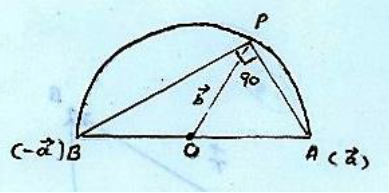# Vector Analysis by Hameed Ullah: NotesNote of vector analysis by Hammed Ullah. These notes are send by Umer Asghar, we are very thankful to him for providing these notes. These notes are for helpful for undergraduate level (BSc or BS).

 Name Notes of vector analysis Mr. Hameed Ullah Mr. Umer Asghar 20+97+56+51 pages PDF (see Software section for PDF Reader) 1.24+3.14+1.76+1.56 mB
• Scalars
• Vectors
• Position vector
• Unit vector
• Null or zero vector
• Resultant vector
• Collinear vector
• Free vector
• Localized vector
• Parallel vector
• Perpendicular vector
• Scalar product or dot product
• Vector product or cross product
• Scalar triple product
• Vector triple product
• Vector function
• Limit of a vector function
• Continuity of vector function
• Differentiation of a vector function
• The vector differential operator